Free Shipping on orders over US\$49.99

# Fourier Series | Basics, Equation, Derivation of Fourier Coefficients

In the early 1800’s, a French Mathematician known as Baron Jean Baptiste Joseph Fourier studied the conduction of heat in different objects and came up with a mathematical analysis on the subject, which later became the famous Fourier Series. There are numerous engineering problems in the fields of Communication, RADAR, Imaging, Audio, etc. that involves the notion of Fourier Seres (and Fourier Transforms, which is a topic for some other time). For instance, all power signals in the field of communication have a close association with Fourier Series while energy signals with Fourier Transforms.

But what exactly is the Fourier Series? What are Fourier Coefficients and how to derive them? What are the applications of Fourier Series? We will find out answers to all these questions in this guide.

## Introduction

When Joseph Fourier introduced his mathematical model on conduction of heat to the Academy of Sciences in Paris in 1807, they criticized and rejected his work. But later, when he published this work in his ‘Théorie analytique de la Chaleur’ (or The Analytical Theory of Heat) in 1822, it became an integral part of engineering.

Even though the original mathematical analysis was a study of conduction of heat in metals, engineers found that they could apply the same technique for other mathematical, engineering and physics problems.

Today, you can see Fourier Series is very important in the fields of Communication (RADAR, Satellite, Wireless in general), Acoustics, Image Processing (Signal Processing in general), Electrical, Optics and many more.

## What is the Fourier Series?

Okay. Enough introduction. Let us now focus on the main question: What is the Fourier Series? Fourier Series is an Infinite Series of a periodic function in terms of Sine and Cosine functions.

Fourier Series of a periodic waveform is an infinite sum sine and cosine waveforms, where the frequency of each wave (sine and cosine) is an integral multiple of the periodic waveform’s fundamental frequency.

Without any mathematical analysis, these statements might sound ridiculous: why are we turning a simple waveform into a complex combination of trigonometric functions?

Actually, the resulting Fourier Series simplifies the process of signal analysis of the main function (or waveform) at different frequencies. We can derive Fourier Series for any periodic or continuous functions or functions with discontinuous values and its derivatives.

Before proceeding further, we need to understand the basic terminology we mentioned earlier: the Periodic Function. A function f(x) is periodic if for a constant T>0, f(x + T) = f(x). Here, T is known as the Period of the function.

Periodic Function: f(x + T) = f(x), T>0

f(x) = f(x +2T) = f(x + 3T) = … T is the period of the function f(x). The function f(x) repeats itself after equal intervals. Trigonometric functions such as sin(x), cos(x), tan(x) etc. are some simple examples of periodic functions.

Now, coming back to the Fourier Series, if f(x) is a periodic function, then we can express it as an infinite sum of sine and cosine functions as follows:Here, a0, an and bn are known as Fourier Coefficients. The values of these coefficients are what define the Fourier Series of a function. Constant a0 is the average value of the periodic function while an and bn are the amplitudes of various sinusoidal functions.

We can calculate a0, an and bn using the following expressions. For example, if f(x) is a periodic function, then Fourier Coefficients of its Fourier Series in the interval T ≤ x ≤ T+2π are as follows: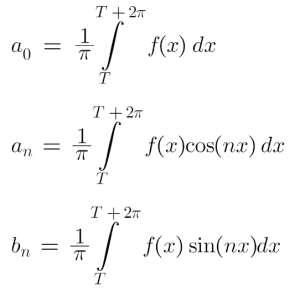The equations of a0, an and bn are known as Euler’s Formulae.

In the previous Fourier Series equation, we used both sine and cosine functions. But we can further modify the equation to give an equation only in terms of sinusoids.

We have the term ancos(nx) + bnsin(nx) in the equation. We can re-write this as follows:Using these terms, we can derive the sinusoid only Fourier Series Expression of a function as: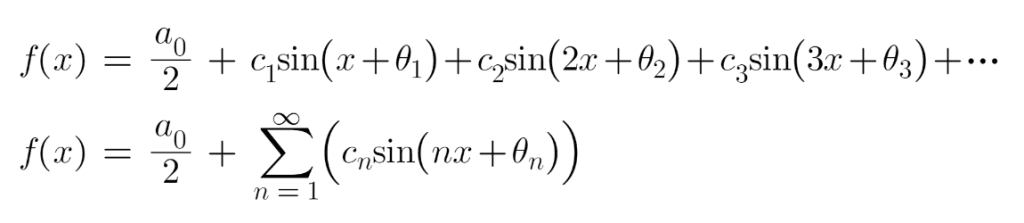In the above equation, notice that for n = 1, the sinusoidal quantity has the same frequency as the main function (which is ‘x’ in this case) and it is the Fundamental Frequency of the main waveform. All the subsequent frequencies (for n = 2, n = 3 and so on) are integral multiples of this fundamental frequency which we call as Harmonic Frequencies.

So, for n = 2, the frequency of the corresponding sinusoid is known as Second Harmonic. Similarly, for n = 3, it is Third Harmonic etc.

## Derivation of Fourier Coefficients

From the above discussion, it is clear that the Fourier Coefficients a0, an and bn are the critical values that we need to calculate for any Fourier Series. We have already seen the expressions for these constants but let us try to derive them.

For this, let us assume that f(x) is a periodic function and its Fourier Series for the interval [T, T+2π] i.e., T ≤ x ≤ T+2π is given by:### Expression for a0

In the above equation, let us integrate both sides from x=T to x=T+2π. We get:From the above equation, we can get the expression for a0 as:### Expression for an

Now, consider the original Fourier Series expression once again. Multiply both sides by ‘cos(mx)’ and integrate the resulting equation from x=T to x=T+2π.In the above expression, if you observe closely, the integrals corresponding to a0 and b (first and third) are always zero. Coming to the second integral corresponding to an, for all m ≠ n cases, it becomes zero and the only possible outcome is for value m = n. Therefore,From the above equation, we can get the expression for an as: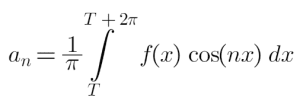### Expression for bn

Now, consider the original Series expression once again. Multiply both sides by ‘sin(mx)’ and integrate the resulting equation from x=T to x=T+2π.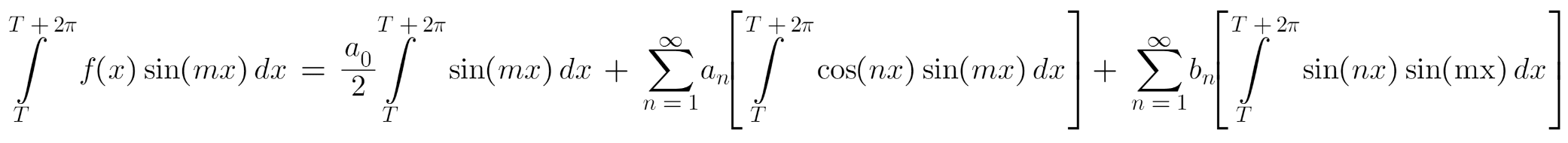In the above expression, the integrals corresponding to a0 and a (first and second) are always zero. Coming to the third integral corresponding to bn, for all m ≠ n cases, it becomes zero and the only possible outcome is for value m = n. Therefore,From the above equation, we can get the expression for bn as: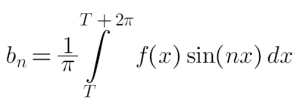## How to Obtain Fourier Series of a Function f(x)?

Peter Gustav Lejeune Dirichlet formulated sufficient conditions that a periodic function must satisfy in order to derive Fourier Series. These are popularly known as ‘Dirichlet’s Conditions’.

We can express any function f(x) in its Fourier Series form as:Where a0, an and bn are constants if the following “sufficient conditions” are satisfied.

• The function f(x) is a periodic, single-valued, well-defined and finite function.
• It has a finite number of discontinuities in any period.
• f(x) has finite number of maxima and minima in a bounded interval.

If these conditions are met, then we can obtain the Fourier Series of any function using the following steps:

Assume the generic form of Series with respect to the function as follows:Calculate the value of a0 using the following formula:Compute the value of an using the following expression:Calculate the value of bn using the following formula:Now, substitute the values of a0, an and b in the original expression (from Step 1) and you have the final Fourier Series of the function f(x).

## Fourier Series Applications

The concept of representing any waveform (or function) purely in terms of sinusoids is a powerful tool in mathematics, engineering (electronics, communication, mechanical, etc.), acoustics, image and video processing.

Fourier Series and Fourier Transforms, which together form a new set of mathematical modelling known as Fourier Analysis, are very important in several scientific applications to solve ordinary and partial differential equations, signal processing, statistics, seismography, oceanography, sonar, cryptography etc.

## Conclusion

This was an introductory guide on Fourier Series. Joseph Fourier developed a mathematical model of conduction of heat in metals, which later became the Fourier Series. In this, we can represent any continuous, periodic function (waveform) purely in the form of sinusoids. We have seen the basic Fourier Series expression, Fourier Coefficients, Dirichlet’s Conditions and many more.

We will be happy to hear your thoughtsEnable registration in settings - general
• Total (0)
0